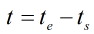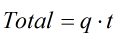# C153 – Totalisation – User Entered Flowrate

## Description

This calculates the total from a user entered flow rate.

Kelton calculation reference C153

KIMS calculation reference K113

## Options

### Flowrates

• Volume
• Mass
• Standard Volume
• Normal Volume
• Energy

This option allows selection of the types of flow rate to be totalised.

### Enter elapsed times as

• Duration
• Start and end time

This option is used to select a method of entering the elapsed.

### Totals

• Use start and end totals

This option is used to input 2 totals and find the incremental total between the start and end totals.

## Calculation

### Calculate the elapsed time

If start and end time is selected for the time input:Where te = The time at the end of the test ts = The time at the start of the test

### Calculate the total

The total is calculated by multiplying the flow rate by the durationWhere t = Duration q = Flow rate

Note: The units will correspond to the flow rate, for example a mass value will be returned for a mass flow rate and a volume returned for a volume flow rate.

Back